Study on Role of Microorganisms in Depolymerization Processes of Xenobiotic Polymers | OMICS International
Journal of Environmental Analytical Chemistry
All submissions of the EM system will be redirected to Online Manuscript Submission System. Authors are requested to submit articles directly to Online Manuscript Submission System of respective journal.

# Study on Role of Microorganisms in Depolymerization Processes of Xenobiotic Polymers

Masaji Watanabe1* and Fusako Kawai2

1Graduate School of Environmental and Life Science, Okayama University, Japan

2Center for Nano materials and Devices, Kyoto Institute of Technology, Japan

*Corresponding Author:
Masaji Watanabe
Graduate School of Environmental and Life Science
Okayama University, Japan
Tel: +81862518829
E-mail: [email protected]

Received Date: February 04, 2014; Accepted Date: November 03, 2014; Published Date: November 05, 2014

Citation: Watanabe M, Kawai F (2014) Study on Role of Microorganisms in Depolymerization Processes of Xenobiotic Polymers . J Environ Anal Chem 2:119. doi: 10.4172/2380-2391.1000119

Copyright: © 2014 Watanabe M, et al. This is an open-access article distributed under the terms of the Creative Commons Attribution License, which permits unrestricted use, distribution, and reproduction in any medium, provided the original author and source are credited

Visit for more related articles at Journal of Environmental Analytical Chemistry

#### Abstract

A microbial depolymerization process of polyethylene glycol is studied with modeling and simulation based on experimental results. Weight distributions before and after cultivation of microbial consortium E1 for two, four, and seven days were introduced into analysis. Inverse problems for a molecular factor and a time factor of a degradation rate were formulated, and those problems were solved numerically. An initial value problem was solved numerically for simulation of an exogenous type depolymerization process. The role of microorganisms in the depolymerization process was interpreted.

#### Abstract

A microbial depolymerization process of polyethylene glycol is studied with modeling and simulation based on experimental results. Weight distributions before and after cultivation of microbial consortium E1 for two, four, and seven days were introduced into analysis. Inverse problems for a molecular factor and a time factor of a degradation rate were formulated, and those problems were solved numerically. An initial value problem was solved numerically for simulation of an exogenous type depolymerization process. The role of microorganisms in the depolymerization process was interpreted.

#### Keywords

Depolymerization process; Polyethylene glycol; Xenobiotic polymers; Biodegradation; Numerical simulation

#### Introduction

Microbial depolymerization processes are categorized into two types, exogenous type processes and endogenous type processes. In an exogenous type depolymeization process, molecules reduce in size through liberation of monomers from their terminals. Polymers subject to exogenous type depolymerization processes include polyethylene (PE). Essential factors of PE biodegradation are gradual weight loss due to β-oxidation, and direct consumption by cells, which led to the scenario; Large PE molecules lose weight through β-oxidation processes until they become small enough to be directly absorbed by cells. A mathematical model based on the scenario was proposed for simulation of exogenous depolymerization processes of PE [1-3]. Polyethylene glycol (PEG) is another polymer depolymerized in exogenous depolymerization processes. PEG is one of polyethers expressed by HO(R-O)n H (PEG: R=CH2CH2) . Biodegradability of PEG has been reported [5,6]. PEG is depolymerized by liberating C2 compounds [7,8]. Techniques developed for the exogenous type depolymerization processes of PE were extended to the exogenous depolymerization processes of PEG. The weight distributions of PEG with respect to the molecular weight before and after cultivation of the microbial consortium E1 were introduced into analysis to solve inverse problems for molecular factors of degradation rates. Initial value problems were solved for the transitions of the weight distribution. The time dependence of degradation rates was taken into consideration . A model proposed for endogenous depolymerization processes was reformulated for the exogenous depolymerizationprocesses of PEG . A mathematical model was proposed for exogenous depolymerization processes of PE , and applied to exogenous depolymerization processes of PEG . Time dependence of degradation rate based on microbial population was considered . In previous studies [13,14], a microbial depolymerization process of PEG 6000 was analyzed. The microbial consortium E1 was cultivated in culture media in which PEG 6000 was the only carbon source, and weight distributions before and after cultivation were compared. Figure 1 shows the weight distributions of PEG before and after cultivation of the microbial consortium E1 for two and four days. Figure 2 shows the weight distribution of PEG after cultivation of the microbial consortium E1 for seven days. Figure 3 shows the transition of residual PEG in the culture medium. Figure 4 shows the transition of optical density of the culture medium. In this study, the microbial depolymerization of PEG 6000 was revisited. The weight distributions before and after cultivation of the microbial consortium E1 for two days were introduced into analysis of an inverse problem for a molecular factor of a degradation rate. A time factor of the degradation rate based on the residual PEG was obtained. An initial value problem was solved for simulation of the transition of the weight distribution and the microbial population.

Figure 1: Weight distributions of PEG 6000 before and after cultivation of the microbial consortium E1 for two and four days . In previ

Figure 2: Weight distribution of PEG 6000 before and after cultivation of the microbial consortium E1 for seven days .

Figure 3: Transition of residual PEG in the culture medium for the molecular weight greater than or equal to 3.7 and less than equal to 4.2.

#### Mathematical Model for Exogenous Type Depolymerization Processes

Molecules of a single polymer all have the same chemical structure, but the degree of polymerization varies from one molecule to another. Let t be the time and M be the molecular weight. Let w (t, M) be the weight distribution with respect to the molecular weight M at time t, which means that the total weight of the molecules of molecular weight M between A and B at time t is given by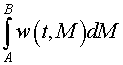For K ≤ M, a molecules with molecular weight K is produced from a molecules with molecular weight M by truncation of a part with molecular weight M − K. Suppose that γ (t, M) is the loss in w (t, M) per unit time and per unit weight, and that q (K, M) is the decrease in w (t, M) per unit weight due to the weight shift from w (t, M) to w (t, K), such that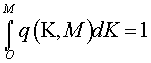(1) The equation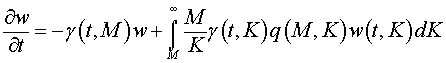(2) Time factors of degradability include temperature, dissolved oxygen, and microbial population. Those factors act evenly on molecules regardless of molecular weight, and the degradation rate γ (t,M) is a product of a function of t, which we denote by σ (t), and a function of M, which we denote by λ (M). In an exogenous depolymerization process, liberation of a monomer is a memoryless process, that is, the amount truncated from a molecule at one stage does not depend on the amount truncated at the previous stage. The probability of a molecule with molecular weight M to become a molecule with molecular weight K is given by an exponential function of M−K. The weigh shift q (K,M) from the class of molecules with molecular weight M to the class of molecules with molecular weight per unit time and unit weight is given by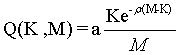where a is independent of K The constant ρ is given by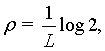where L is the molecular weight of a monomer, that is, L = 44 (CH2CH2O) . Condition (1) leads to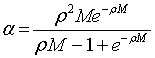Substitution of γ(t,M)=σ(t)λ(M)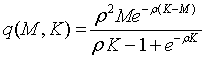in to equation (2) results in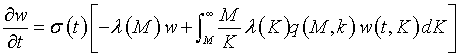(3) Where,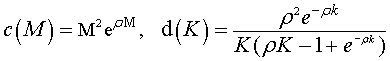(4) The only time factor in the microbial depolymerization process depicted by the Figures 1 and 2 was the microbial population. A mathematical model for the transition of microbial population was proposed in a previous study . In this study, the carbon source A (t) for the microorganism at time t is the residual polymer in the culture medium at time t,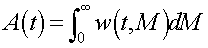(5) Let h be the amount of the carbon source, for which a unit microbial population is stationary. The microbial population σ (t) grows over a period in which h < A (t) /σ (t), and decreases over a period in which h > A (t) /σ (t). The growth rate of the microbial population is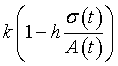for some positive constant k, and the microbial population σ (t) is a solution of the equation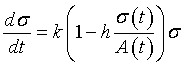(6) Suppose that an initial weight distribution f (M) and the initial microbial population σ0 are given. Equations (3) and (6) and the initial conditions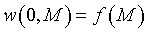(7) and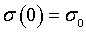(8) form an initial value problem.

#### Numerical Solution of Inverse Problem for the Molecular Factor of the Degradation Rate

The initial value problem (3), (6), (7), (8) is solvable provided the time factor σ (t) and the molecular factor λ (M) of the degradation rate are prescribed. In order to find those factors, consider the change of variables from t to τ, where τ is given by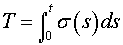(9) Let W (τ, M) = w (t, M). The time factor is removed from equation (3), and it now becomes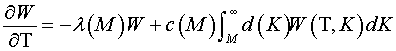(10) Given the molecular factor of the degradation rate λ (M), equation (10) and the initial condition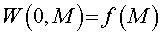(11) form an initial value problem. Equation (10), the initial condition (11) and the condition for τ = T,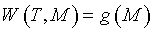(12) form an inverse problem for the molecular factor of the degradation rate λ (M), for which the solution of the initial value problem (10) and (11) also satisfies the condition (12). Techniques to solve the inverse problem for the molecular factor of the degradation rate were developed in previous studies [10,12]. The weight distributions before and after cultivation of the microbial consortium E1 for two days (Figure 1) were set for the initial weight distribution and the final weight distribution, respectively, and the inverse problem (10), (11), (12) was solved numerically for the molecular factor of the degradation rate for the functions c (M) and (K) given by the expressions (4) (Figure 5). Once the time factor of the degradation rate λ (M) was found, the initial value problem (10),(11) was solved. Figure 6 shows the graph of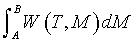(13) which corresponds to the residual PEG in the culture medium, Note that the results shown in the Figures 3 and 6 show that t=2 and t=4 correspond to τ=2.01496739 and τ=8.77024551, respectively.

Figure 5: Degradation rate based on the weight distributions before and after cultivation of the microbial consortium E1 on PEG 6000 for two days.

Figure 6: Graph of the integral (13), where W (t) is the solution of the initial Value problem (10) and (11).

#### Numerical Simulation for Depolymerization Process of PEG 6000

The initial value problem (3),(6),(7),(8) was solved numerically for another set of experimental data for various values of parameters which appear in equation (6), where A (t) was given by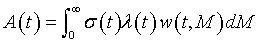(14) for some constants a and b, techniques to determine the parameters σ0, k, and h were proposed in a previous study . The inverse problem was solved for the PEG 6000 with the molecular factor based on the weight distribution before and after cultivation of the microbial consortium E1 for two days , and the weight distributions after cultivation for two and four days . Suppose that S (τ) = σ (t). The definition of τ by equation (9) implies that S (τ) is the solution of the initial value problem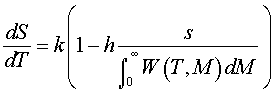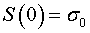Let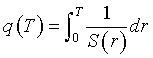Note that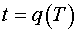holds. Define the functions of σ0, k, and h by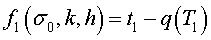,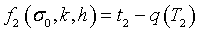(16) Where t1 = 2, t2 = 4, τ1 = 2.01496739, and τ2 = 9.01763865. Equations based on the results shown in Figures 3 and 6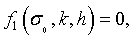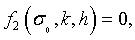(17) hold. Intervals [σ0min, σ0max],[kmin, kmax],[hmin, hmax] were set to solve the system of equations numerically, where σ0min = 0.4 , σ0max = 0.6, kmin = 0.6, kmax = 0.7 , hmin = 5.0 , hmin = 7.0 Those intervals are discretized evaluate gi0, k, h) (i=1, 2, 3) for the following Values of the parameters.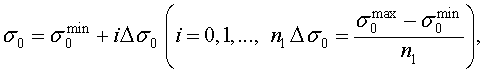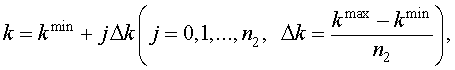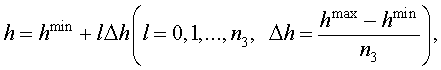where n1 = 50, n2 = 50, n3 = 50. The values of the parameters σ0 = 0.492,k = 0.656,h = 5.88 that minimized the error: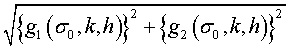(20) were found in the set of the parameter values (18). The error (20) was 0.000363189884 for the values (19). Values (19) were assigned to the parameters were used to solve the initial value problem; the numerical results are shown in Figures 7-9.

Figure 7: A numerical result for the weight distribution after cultivation of the microbial consortium E1 for two and four days. Experimental results are also shown.

#### Conclusion

Figures 3 and 4 show that viable cells increased under su?cient supply of the carbon source and started decreasing when it became insu?cient. Figure 8 shows the transition of the viable population. It shows that the viable population was on an increasing phase for the ?rst ?ve days, and that it was on a decreasing phase for the next two days. The microbial consortium E-1 consists of S. terrae and Rizobium sp. S. terrae plays the major role as the degrader in microbial depolymerization processes of PEG . The viable population shown in the ?gure 8 corresponds to S. terrae. In an early stage of the depolymerization process, S. terrae are dominant in the microbial population. Suppose that a linear relation holds between the viable cells and the optical density. The linear function was determined using the microbial population and the optical density before and after cultivation of microbial consortium E-1 for two days. Figure 10 shows the graph of the microbial population obtained from the result shown in the Figure 8 with the linear function. Figure 10 also shows the measured optical density. Some cells lose viability and remain in the culture medium. In the cultivation of microbial consortium E-1, Rizobium sp. starts to be dominant as S. terrae starts decreasing. Those facts led to the result that measured optical density after cultivation for seven days was higher than the optical density obtained by the simulation.

#### Acknowledgment

The authors would like to thank Ms Y. Shimizu for her technical support. This work was supported by JSPS KAKENHI Grant Number 24540127.

#### References

Select your language of interest to view the total content in your interested language

### Article Usage

• Total views: 12485
• [From(publication date):
January-2015 - Nov 17, 2019]
• Breakdown by view type
• HTML page views : 8660Can't read the image? click here to refresh